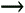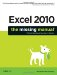# Section 12.4. Controlling Recalculation

12.4. Controlling Recalculation

Ordinarily, Excel recalculates a formula whenever you change any of the cells the formula uses, and whenever you open the worksheet containing the formula. This behavior is extremely convenient because it ensures that your information never gets out of date. However, it can cause trouble if your worksheet contains time-consuming calculations or extremely large tables of data. In this situation, life can slow to a crawl while Excel recalculates and updates all its formulas.

Tip: You'll know the recalculation is at work when the mouse pointer changes to an hourglass and the word Recalc (followed by the number of cells left to be recalculated) appears on the left side of the Formula bar. Even though your cursor looks like an hourglass, you can still enter data and use the menu, but every time you perform any of these activities, it briefly pauses the recalculation process.

With the exponential increase in computer speed (and Microsoft's minor tweaking of Excel's performance), large worksheets aren't the problem they used to be. However, some Excel aficionados still report problems when crunching data that's thick with formulas. If you're using Excel to plot the path of orbital satellites or to analyze census data, you may be interested in switching off Excel's automatic calculation to make your worksheet more responsive .

1. Select ToolsOptions.

The Options dialog box appears.

2. Select the Calculation tab.

The Calculation tab provides a slew of options. The first three radio buttons (Automatic, Automatic except tables, and Manual) are the most important.

3. To switch to manual calculation, click the Manual radio button.

The "Automatic except tables" option still uses automatic calculation, but it doesn't recalculate data tables. (Data tables were discussed earlier in this chapter.)

4. If you don't want Excel to perform a recalculation before saving the workbook, turn off the "Recalculate before save" checkbox.

In a complex worksheet, leaving this option on could increase the amount of time it takes to save your data. However, it also ensures that the next time you open the file, you start with freshly updated data.

5. Click OK.

In manual recalculation mode, Excel calculates most simple formulas when you enter them. However, if you modify cells that the formula uses, the program doesn't recalculate the formulainstead, it keep displaying the old result. If you see the word Calculate in the status bar, that's your clue that some data has changed and a recalculation is a good idea to refresh your formula results.

6. You can press F9 to recalculate all the formulas in the workbook at any time. You can also select ToolsOptions again, click the Calculation tab, and use the Calc Now or Calc Sheet buttons. The Calc Sheet button calculates only the current worksheet, not the entire workbook.

Note: Unfortunately, the recalculation settings are application-wide. That means if you switch off automatic calculation for one worksheet, it affects any other worksheets you're using. If you don't remember that you've switched off automatic recalculation, you may end up using and printing stale data.Excel 2010: The Missing Manual
ISBN: 1449382355
EAN: 2147483647
Year: 2003
Pages: 185